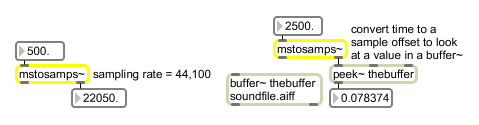# mstosamps~

Convert milliseconds to samples

## Description

mstosamps~ will convert an incoming signal carrying a millisecond value and output a signal which converts those milliseconds to a number of samples (at the current sampling rate).

None.

## Messages

 int millisecond-value [int] Millisecond values received in the inlet are converted to a number of samples at the current sampling rate and sent out the object's right outlet. The output might contain a fractional number of samples. For example, at 44.1 kHz sampling rate, 3.2 milliseconds is 141.12 samples. float millisecond-value [float] Millisecond values received in the inlet are converted to a number of samples at the current sampling rate and sent out the object's right outlet. The output might contain a fractional number of samples. For example, at 44.1 kHz sampling rate, 3.2 milliseconds is 141.12 samples. signal Incoming millisecond values in the signal are converted to a number of samples at the current sampling rate and output as a signal out the mstosamps~ object's left outlet. The output may contain a fractional number of samples.

## Output

float: Out right outlet: The number of samples corresponding to the millisecond value received as a float or int in the inlet.
signal: Out left outlet: The number of samples corresponding to the millisecond values in the input signal.

## ExamplesTime expressed in milliseconds comes out expressed in samples